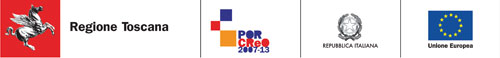# CODING

To define the coding p
rocess the already existing Data Matrix standard (standard ISO/IEC16022:2006) was used as a starting point. The first step was to define the set of characters of interest and the number of colors to be used. Introducing additional colors for the composition of the board entails increasing the dimension of the base from n=2 to a larger n, equal to the number of colors, thus increasing the depth of the code. This implies that the code itself occupies a smaller area and for a given surface it allows storage of a quantity of information that grows exponentially with the size of the base. The following table reports the number of combinations that can be realized with a 8-bit string depending on the dimension of the base:

 N° di bit 8 Base 2 3 4 5 Combinations 256 6561 65536 390625

As an alternative way of considering the problem, the next table shows the number of bits required for coding 256 characters as a function of the dimension of the base:

 Base 2 3 4 5 Bit needed /256 characters 8 6 4 4

The process of development started by defining a coding protocol that used 5 colors: this choice was aimed at building a code that could adequately condense the information without becoming excessively complex to realize and even more to decode. To assign a numerical value to each character we have taken as reference the ASCII code.
The ASCII (American Standard Code for Information Interchange) is a character-encoding scheme originally based on the English alphabet.
The ASCII is a 7 bit coding system (or better 8 bit, as 1 bit is used for the parity check aimed at detecting possible errors) commonly used in computers. It was originally proposed by IBM engineer Bob Bemer of IBM in 1961, and subsequently accepted as a standard by ISO (ISO 646). The initial specification based on 7- bit codes was followed over the years by many proposals to extend it to 8 bits, with the aim of doubling the number of characters represented. Actually Computers use one of these extensions, that has become a standard and is called extended ASCII. In this extended ASCII, the characters added are accented vowels, semi-graphic symbols and other less common symbols. The extended ASCII characters are encoded in the so-called codepage. Alla specifica iniziale basata su codici di 7 bit fecero seguito negli anni molte proposte di estensione ad 8 bit, con lo scopo di raddoppiare il numero di caratteri rappresentabili. Nei pc si fa per l'appunto uso di una di queste estensioni, ormai standard di fatto, chiamata extended ASCII. In questo ASCII esteso, i caratteri aggiunti sono vocali accentate, simboli semigrafici e altri simboli di uso meno comune. I caratteri extended ASCII sono codificati nei cosiddetti codepage.
The ASCII code is the starting point of the coding algorithm of the n-bit DataMatrix. The sequence of operations performed by the algorithm of encoding is as follows:

• the string to decode is divided into single characters

• ach character is associated to its ASCII code by the software

• this code is used as an index to track a custom code linked to the character, in a text file created to this purpose
• this custom code is converted from the decimal base to the chosen coding base ( which depends on the number of colors used), using a word (a fixed size group of bits that are handled as a unit) of a number of bits adequate to represent the code
• control bits are added to the obtained code
• finally, colored squares are created by converting the digits of the obtained number according to the specifically set match Digit=Color
• these squares are then inserted into a chessboard (whose corners satisfy the characteristics needed for the recognition and proper positioning of the scheme during the reading phase for subsequent decoding), ordered according to an interleaving algorithm.

As we mentioned above, the encoding of all ASCII characters using a base of size 5 requires the use of a 4 bits word. The error correction algorithm performs the partial sums of the code bits and the addition of 3 control bits for each character.
In doing so the code length has to be doubled and that would mean reducing the avaiable space, and hence the amount of information contained in a given area, and at the same time doubling the chance of making mistakes in the hope of correcting one. The evaluation of these aspects has led us to the decision of changing the coding system and reducing the codable charset. A software routine generates a set of 5 bits codes in base 5. Among the obtained codes, another routine selects only the ones that differ for at least 3 digits in given positions. In that way the set of codes obtained consists of 74 units, which are sufficient to encode all the letters, both uppercase and lowercase, numbers and the most frequently used punctuation symbols.
Using the same system of comparison as for 7 bit codes, the software is able to detect and correct all single errors and detect double errors. In this way, by reducing the set of characters to the essential ones, it is also possible to reduce the length of the word by 28% while maintaining the same number of correctable errors (and increasing it in percentage from 14% to 20%), while still having an extremely versatile set of symbols.Intervento cofinanziato POR CReO FESR 2007-2013 Linee 1.5 e 1.6

Sinerlab s.r.l. | Via Trento 12 - 51039 – Quarrata (PT) | Tel. e Fax 0573 73039 www.sinerlab.it